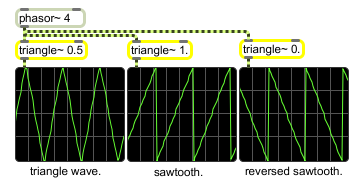# triangle~

Triangle/ramp wavetable

## Description

triangle~ is a triangle/ramp wavetable with signal input to change phase offset of the peak value. The default lo/hi points are -1. and 1., but may be changed using the lo/hi messages.

## Arguments

Name Type Opt Description
peak-value-phase-offset float opt Peak value phase offset, expressed as a fraction of a cycle, from 0 to 1.0. The default is 0.5. A value of 0 produces a ramp waveform when the triangle~ object is driven by a phasor~, and a value of 1.0 produces a sawtooth waveform.

## Messages

 float triangular-control [float] In left inlet: Any signal, float, or an input signal progressing from 0 to 1 is used to scan the triangle~ object's wavetable. The output of a phasor~ or some other audio signal can be used to control triangle~ as an oscillator, treating the contents of the wavetable as a repeating waveform. In right inlet: Peak value phase offset, expressed as a fraction of a cycle, from 0 to 1.0. The default is 0.5. Scanning through the triangle~ object's wavetable using output of a phasor~ with a phase offset value of 0 produces a ramp waveform, and a phase offset of 1.0 produces a sawtooth waveform. hi maximum-value [float] In left inlet: The word hi, followed by an optional number, sets the maximum value of triangle~ for signal output. The default value is 1.0. lo minimum-value [float] In left inlet: The word lo, followed by an optional number, sets the minimum value of triangle~ for signal output. The default value is 1.0. signal In left inlet: Any signal, float, or an input signal progressing from 0 to 1 is used to scan the triangle~ object's wavetable. The output of a phasor~ or some other audio signal can be used to control triangle~ as an oscillator, treating the contents of the wavetable as a repeating waveform. In right inlet: Peak value phase offset, expressed as a fraction of a cycle, from 0 to 1.0. The default is 0.5. Scanning through the triangle~ object's wavetable using output of a phasor~ with a phase offset value of 0 produces a ramp waveform, and a phase offset of 1.0 produces a sawtooth waveform.

## Output

signal: A signal which corresponds to the value referenced by the triangle~ object's input signal. If the output of a phasor~ or some other audio signal is used to scan the triangle~ object, the output will be a periodic waveform.

## Examplestriangle~ lets you generate ramping waveforms with different reversal points